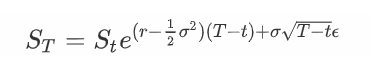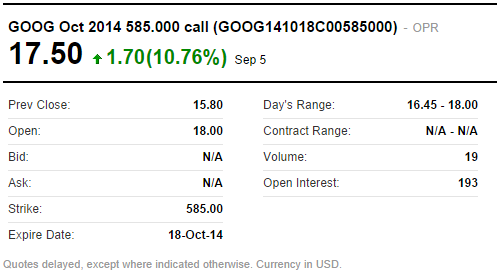# 使用蒙特卡罗模拟期权定价## 期权，及其价值

CT=max(0,ST−K)

PT=max(0,K−ST)## 风险中性估值

Ct=PV(E[max(0,ST−K)])

Pt=PV(E[max(0,K−ST)])## 模拟资产价格

Black Scholes 模型中使用的股票价格行为模型假设我们有一个已知的波动性，我们有一个无风险利率，并且资产的价格遵循几何布朗运动。为此，我们需要知道：

r 是我们要贴现的无风险利率。 σ 是波动率，即股票回报的年化标准差。 (T-t) 给了我们年化的到期时间。 例如，对于 30 天选项，这将是 30/365=0.082… S 是在时间 t 标的资产的价格。 ϵ 是我们的随机值。 它的分布必须是标准正态（均值为 0.0，标准差为 1.0）

## 期权定价

1.3620 120.64 10.64 10.58
-0.7784 89.13 0.00 0.00
-0.9408 87.11 0.00 0.00
0.2227 102.69 0.00 0.00
-0.0364 98.99 0.00 0.00
-1.4303 81.28 0.00 0.00
-0.8306 88.47 0.00 0.00
1.5155 123.28 13.28 13.21
-1.5679 79.71 0.00 0.00
-1.6718 78.55 0.00 0.00

## 为真实期权定价```def generate_asset_price(S,v,r,T):
return S * exp((r - 0.5 * v**2) * T + v * sqrt(T) * gauss(0,1.0))```

```S = 857.29 # underlying price
v = 0.2076 # vol of 20.76%
r = 0.0014 # rate of 0.14%
T = (datetime.date(2013,9,21) - datetime.date(2013,9,3)).days / 365.0

print generate_asset_price(S,v,r,T)
>>> 862.1783726682384```

```def call_payoff(S_T, K):
return max(S_T - K, 0.0)

print call_payoff(862.18, 860)
>>> 2.1799999999```

## 完整的模拟

```import datetime
from random import gauss
from math import exp, sqrt

def generate_asset_price(S,v,r,T):
return S * exp((r - 0.5 * v**2) * T + v * sqrt(T) * gauss(0,1.0))

def call_payoff(S_T,K):
return max(0.0,S_T-K)

S = 857.29 # underlying price
v = 0.2076 # vol of 20.76%
r = 0.0014 # rate of 0.14%
T = (datetime.date(2013,9,21) - datetime.date(2013,9,3)).days / 365.0
K = 860.
simulations = 90000
payoffs = []
discount_factor = math.exp(-r * T)

for i in xrange(simulations):
S_T = generate_asset_price(S,v,r,T)
payoffs.append(
call_payoff(S_T, K)
)

price = discount_factor * (sum(payoffs) / float(simulations))
print 'Price: %.4f' % price```

`Price: 14.5069`## 往期干货分享推荐阅读

Omega System Trading and Development Club内部分享策略Easylanguage源码

AMA指标原作者Perry Kaufman 100+套交易策略源码分享

【数量技术宅|金融数据分析系列分享】为什么中证500（IC）是最适合长期做多的指数

【数量技术宅|金融数据分析系列分享】如何正确抄底商品期货、大宗商品

【数量技术宅|量化投资策略系列分享】股指期货IF分钟波动率统计策略

【数量技术宅 | Python爬虫系列分享】实时监控股市重大公告的Python爬虫